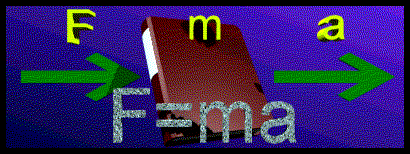# The Physics Department - Mechanics, ForcesA force can most easily be described as a push or a pull. When a force is applied to an object, the velocity of that object changes. This change in velocity constitutes an acceleration. So, forces are tied to accelerations. Galileo and Newton were scientists who developed our understanding of forces.

 Galileo and the Concept of Inertia Forces in General Net Force Proportions Isaac Newton's Three Laws of Motion Components of a force vector Gravity The inclined plane Torque General Problems

An understanding of forces should include an understanding of vectors.

Custom Search